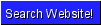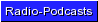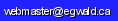Egwald Statistics: Multiple Regression Linear and Restricted Multiple Regression by Elmer G. Wiens Egwald's popular web pages are provided without cost to users. Please show your support by joining Egwald Web Services as a Facebook Fan:Follow Elmer Wiens on Twitter:Researchers in the physical and social sciences often want to determine a relationship among a number of variables based on a set of data that are observations or measurements of these variables over a number of instances. Usually, one variable is called the dependent variable while the other variables are independent variables. A linear regression tries to determine the dependent variable as a linear function of the independent variables. Often researchers transform the variables, as in the Cobb-Douglas example below, before they estimate the linear function. While this terminology suggests a causal link from the independent variables to the dependent variable, this can not be inferred by the linear regression techniques alone. Linear regression is also called multiple regression, or least squares estimation. I suggest you step through my examples before you use my package for your own regression study. A. Multiple regression analysis package.   a. Linear multiple regression.       1. Example: Predicting Canadian Elections using the equation:             C = ß0 + ß1 * E + ß2 * OQ + ß3 * P + ß4 * BC                 where             C = Percent of seats of Canada taken by the winning party             E = Percent of seats of Eastern Canada taken by the winning party             OQ = Percent of seats of Ontario and Quebec taken by the winning party             P = Percent of seats of Prairie Provinces taken by the winning party             BC = Percent of seats of B.C. taken by the winning party       i. Example: Predicting Canadian Elections using data for the years 1949-1997. These 16 elections yield the equation:             C = -21.691 + 0.117 * E + 0.937 * OQ + 0.136 * P + 0.087 * BC       ii. Example: Predicting Canadian Elections using data for the years 1949-2000. These 17 elections yield the equation:             C = -19.972 + 0.115 * E + 0.903 * OQ + 0.132 * P + 0.103 * BC       (including the 2000 Canadian Election renders the regression coefficient for BC statistically significant)       iii. Example: Predicting Canadian Elections using data for the years 1949-2004. These 18 elections yield the equation:             C = -17.321 + 0.13 * E + 0.857 * OQ + 0.117 * P + 0.113 * BC               (Each regression coefficient is statistically significant)       2. Do your own regression study.   b. Restricted linear regression.       1. Example: Cobb Douglas Production Function       2. Do your own restricted regression study B. 1. Check out the derivation of the regression model      2. Statistics, multiple regression, and numerical analysis references.       3. Linear Algebra background to least squares (multiple regression). C. Learn key concepts from the theory of probability used in statistics and regression analysis: Probability and Stochastic Processes. D. In my internet essay on government enterprises and democracy, I use the regression equation from A. to analyze how the Canadian Government handles its Crown corporations.Copyright © Elmer G. Wiens:   Egwald Web ServicesAll Rights Reserved.    Inquiries